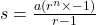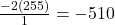## Find the sum of the geometric series given a1=−2, r=2, and n=8. A. -510 B. -489 C. -478 D. 2

Question

Find the sum of the geometric series given a1=−2, r=2, and n=8.
A. -510
B. -489
C. -478
D. 2

in progress 0
6 months 2021-07-16T20:47:54+00:00 1 Answers 62 views 0

## Answers ( )

A. -510

Step-by-step explanation:

We are given the variable values:

• a = -2
• r = 2
• n = 8

Geometric series formula:Plugging in values we have:Simplifying the equation we are left with: